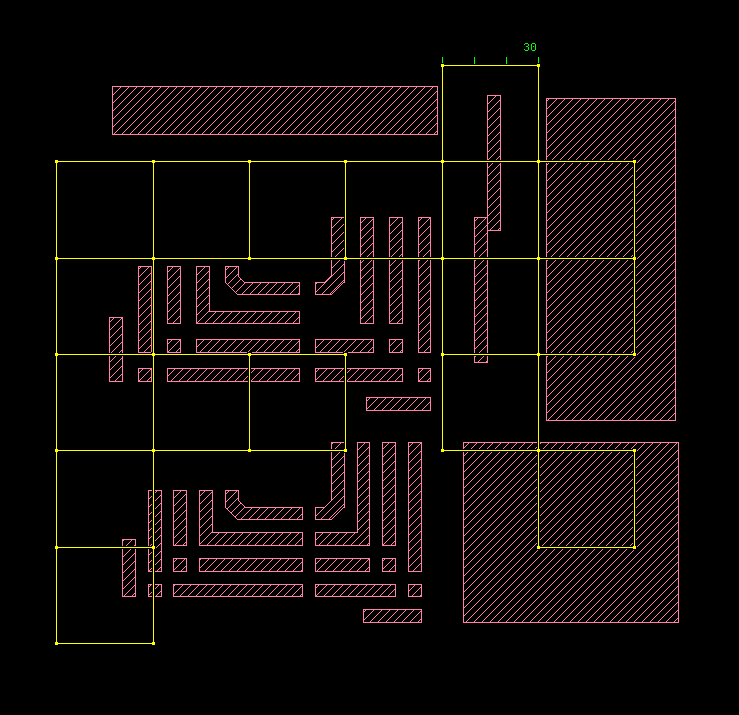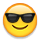# Density DRC checks

Foundry DRC decks often include density checks. Calibre decks often define this as density in regions of a certain size.
Although I found some topics discussing density it's not clear to me how to best handle density checks in a klayout drc script.
I would like them to be part of a drc script that can be run in batch.

• Hi,

there is no out-of-the-box DRC command yet, but a bare metal feature is there (the TilingProcessor class). You can wrap it inside your DRC script like this:

``````# Provides a density check feature: include this code at the beginning of your DRC script

def dens_check(output, input, tile_size, threads, min, max)

tp = RBA::TilingProcessor::new

tp.tile_size(tile_size, tile_size)
tp.output("res", output.data)
tp.input("input", input.data)
tp.dbu = 1.dbu  # establish the real database unit
tp.var("vmin", min)
tp.var("vmax", max)
tp.queue("_tile && (var d = to_f(input.area(_tile.bbox)) / to_f(_tile.bbox.area); (d < vmin || d > vmax) && _output(res, _tile.bbox))")
tp.execute("Density check")

end

# -- this is the actual DRC script --

report("Metal density DRC check")

metal = input(1, 0)

# that is one use case. It checks between 10% and 90% on 30µm tiles and with 4 CPU's:
dens = polygon_layer
dens_check(dens, metal, 30.um, 4, 0.1, 0.9)

dens.output("Dens", "Metal density between 10 and 90%")
``````

Matthias

• I was rummaging around in some of the examples repositories
and I ran across two macro scripts which, together, look like
they might do a density check:

cell_bbox.lym gives the bounding box info for the layout
calc_area_hier.lym gives the total area of a specified layer

So you could (I guess) stack 'em up, harvest the output and
do the arithmetic to get your density numbers. I'd assume
they could be put all together in a "check 'em all" macro
(one bounding box, and each layer of interest). Limit check
I suppose would be another effort.

But looks like there's a cleaner approach in the works.

• Hi Matthias,

This is cool! Can it be adapted for overlapping tiles, let's say you want to check the density in 30um tiles which overlaps 5um with neighboring tiles. I tried with "tile_border" but no idea how to reflect it in tp.queue:

def dens_check(output, input, tile_size, tile_overlap, threads, min, max)

tp = RBA::TilingProcessor::new

tp.tile_size(tile_size-2.0tile_overlap, tile_size-2.0tile_overlap)
tp.tile_border(tile_overlap, tile_overlap)
tp.output("res", output.data)
tp.input("input", input.data)
tp.dbu = 1.dbu # establish the real database unit
tp.var("vmin", min)
tp.var("vmax", max)
tp.queue("_tile && (var d = to_f(input.area(_tile.bbox)) / to_f(_tile.bbox.area); (d < vmin || d > vmax) && _output(res, _tile.bbox))")
tp.execute("Density check")

end

Cheers,

Tomas

• edited February 2021

Hi Tomas,

I think you just need to enlarge the _tile.bbox inside the "queue" script: "_tile.bbox.enlarged(overlap, overlap)". Or better:

``````tp.tile_border(tile_overlap, tile_overlap)
tp.var("overlap", tile_overlap)
...
tp.queue(<<"SCRIPT"
_tile && (
var bx = _tile.bbox.enlarged(overlap, overlap);
var d = to_f(input.area(bx)) / to_f(bx.area);
(d < vmin || d > vmax) && _output(res, bx)
)
SCRIPT
)
``````

(disclaimer: not tested)

Matthias

• Hi Matthias,

I tried to test it. I had to leave out <<"SCRIPT" and SCRIPT to make the script run (???). The marker browser results are shown in the screenshot below. I would have expected 40um overlapping boxes instead of 30um touching boxes???

Cheers,

Tomas

def dens_check(output, input, tile_size, tile_overlap, threads, min, max)

tp = RBA::TilingProcessor::new

tp.tile_size(tile_size, tile_size) # -2.0*tile_overlap
tp.tile_border(tile_overlap, tile_overlap)
tp.output("res", output.data)
tp.input("input", input.data)
tp.dbu = 1.dbu # establish the real database unit
tp.var("vmin", min)
tp.var("vmax", max)
tp.var("overlap", tile_overlap)
tp.queue("
_tile && (
var bx = _tile.bbox.enlarged(overlap, overlap);
var d = to_f(input.area(bx)) / to_f(bx.area);
(d < vmin || d > vmax) && _output(res, bx)
)
")
tp.execute("Density check")

end

report("Density DRC check")

Layer01 = input(1,0)

Layer01_output = polygon_layer

dens_check(Layer01_output, Layer01, 30.um, 5.0.um, 4, 0.2, 0.8)

Layer01_output.output("Density", "Density between 20 and 80%")• edited February 2021

As I said, not tested ...I think the code had three issues: First the syntax of the inline document. Second the translation of the overlap value into DBU units. And third, the "clip" flag needs to be set to "false" in "_output".

Here is an improved (tested) version:

``````def dens_check(output, input, tile_size, tile_overlap, threads, min, max)

tp = RBA::TilingProcessor::new

tp.tile_size(tile_size, tile_size) # -2.0*tile_overlap
tp.tile_border(tile_overlap, tile_overlap)
tp.output("res", output.data)
tp.input("input", input.data)
tp.dbu = 1.dbu # establish the real database unit
tp.var("vmin", min)
tp.var("vmax", max)
tp.var("overlap", tile_overlap / tp.dbu)
tp.queue(<<"SCRIPT")
_tile && (
var bx = _tile.bbox.enlarged(overlap, overlap);
var d = to_f(input.area(bx)) / to_f(bx.area);
(d < vmin || d > vmax) && _output(res, bx, false)
)
SCRIPT
tp.execute("Density check")

end

``````

Matthias

• Hi Matthias,

It works great, thanks! You are the best.Cheers,

Tomas